# AP Calculus BC Practice Test 43

### Test Information10 questions20 minutes

Calculator Disallowed

1.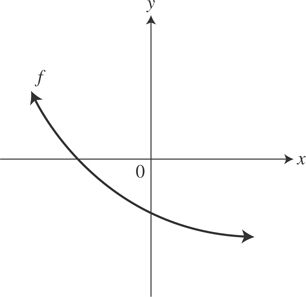The graph of f is shown above, and f is twice differentiable. Which of the following has the largest value?

I. f(0)

II. f′ (0)

III. f″ (0)

2. .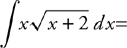3. .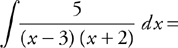4.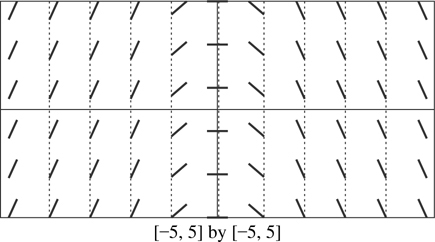A slope field for a differential equation is shown above. Which of the following could be the differential equation?

5. Which of the following is a Taylor series for xe2x about x = 0 ?

6. If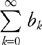converges and 0 < akbk for all k, which of the following statements must be true?

7. If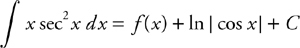, then f(x)=

8. A solid has a circular base of radius 1. If every plane cross section perpendicular to the x-axis is a square, then the volume of the solid is

9. If n is a positive integer, then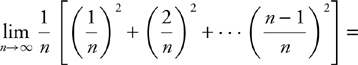10. The area enclosed by the parabola y = x - x2 and the x-axis is revolved about the x-axis. The volume of the resulting solid is